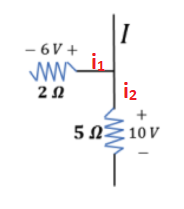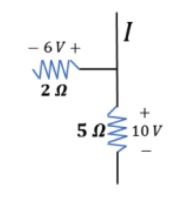# Problem: In the picture below, what is the current in the wire above the junction? Does charge flow toward or away from the junction?

###### FREE Expert Solution

Ohm's law:

$\overline{){\mathbf{V}}{\mathbf{=}}{\mathbf{i}}{\mathbf{R}}}$Looking at the diagram, the wire above the junction supplies current to the two resistors. We know this by observing the positive side of the resistors. Current enters a resistor at its positive sde.

Using Kirchhoff's junction rule:

i = i1 + i2

98% (336 ratings)###### Problem Details

In the picture below, what is the current in the wire above the junction? Does charge flow toward or away from the junction?